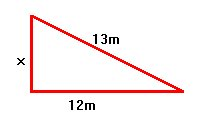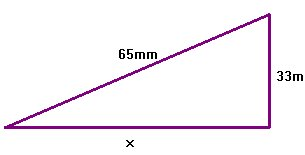WORKSHEET: PYTHAGORAS

Worksheets Descargar PDF
1) Find the missing length in the following right triangles. Round the solution to the nearest hundredth (if necessary)
 a)Solution
 b)Solution
 c)Solution
 d)Solution
2) The legs of an isosceles right triangle are 7 cm long. Find the length of the hypotenuse. Round to the nearest tenth.

Solution
3) Calculate the perimeter of the following figures:
 a)Solution
 b)Solution
 c)Solution
 d)Solution
 e)Solution